# MATLAB Supplementary lecture:

## Math 222 Fall 2021

### For loops and recurrence relations

Any formula which generates a sequence and gives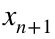in terms of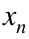is called a recurrence relation. Of course such a formula needs an initial value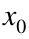. A very simple recurrence relation isThis define a sequence of valuesWe know, from Math 111, that the exact solution to this recurrence isso we plot this as well;
N = 10;
n=0:N; % note that this starts from n=0
y = zeros(1,N+1);
y(1) = 0;
for j=1:N
y(j+1) = y(j) + j;
end
yExact=n.*(n+1)/2; % Note the use of .* to multiply arrays!
plot(n,y,'o',n,yExact,'x')
xlabel('n');ylabel('x')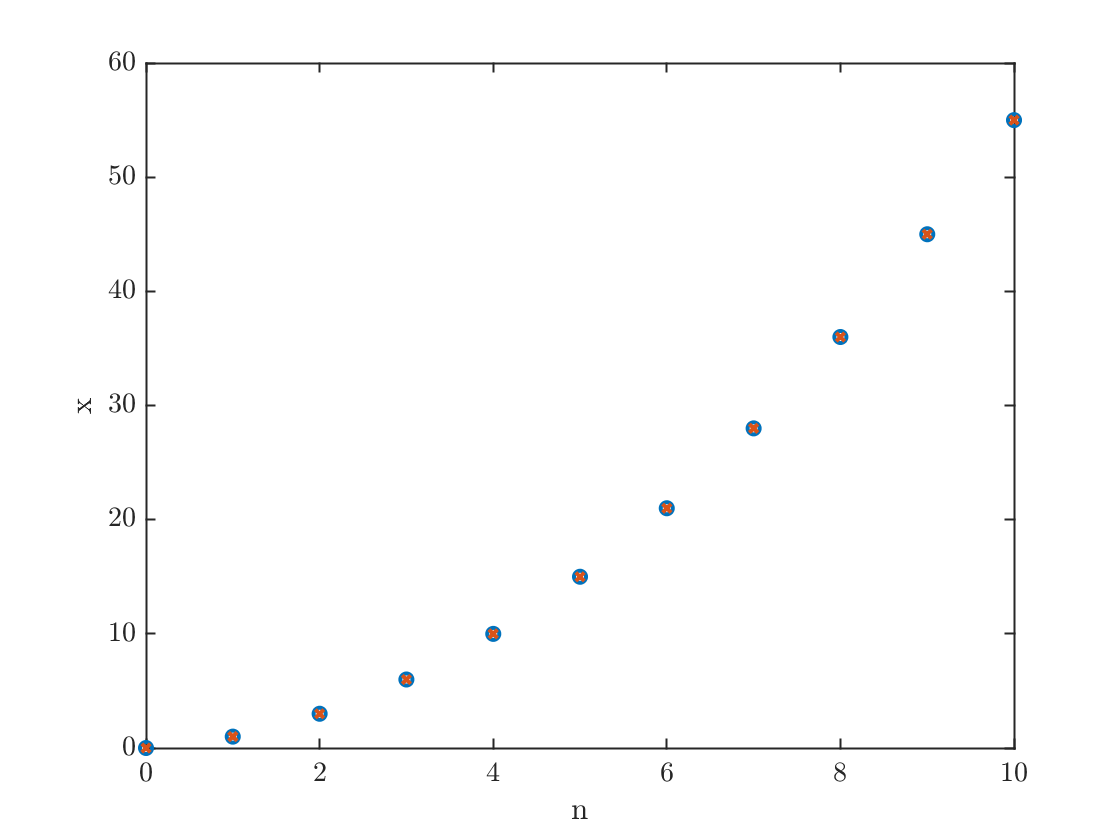One idea not taught in the Onramp: I have plotted both x and xExact in a single plotting command. I think the syntax for this should be obvious once you've seen it.

MATLAB has lots of built-in functions like sin, exp, and abs, for defining the sine, exponential and absolute value functions, but to build a proper Euler method program, we need to be able to define our own.
Here's an example of a function definition. The normal distribution with mean μ and variance σ is defined aswhich we can put into a function as
function y = gaussian(x,mu,sigma)
y = exp(-1/2 * ( (x-mu)/sigma).^2) / sigma / sqrt(2*pi);
end
(The above three lines are only example code, but they are repeated at the bottom of this live script, and those lines work.)
We can call this with the command
y=linspace(-3,3);
mu = 1.5;
sigma=1;
y= gaussian(y,mu,sigma);
plot(y,y)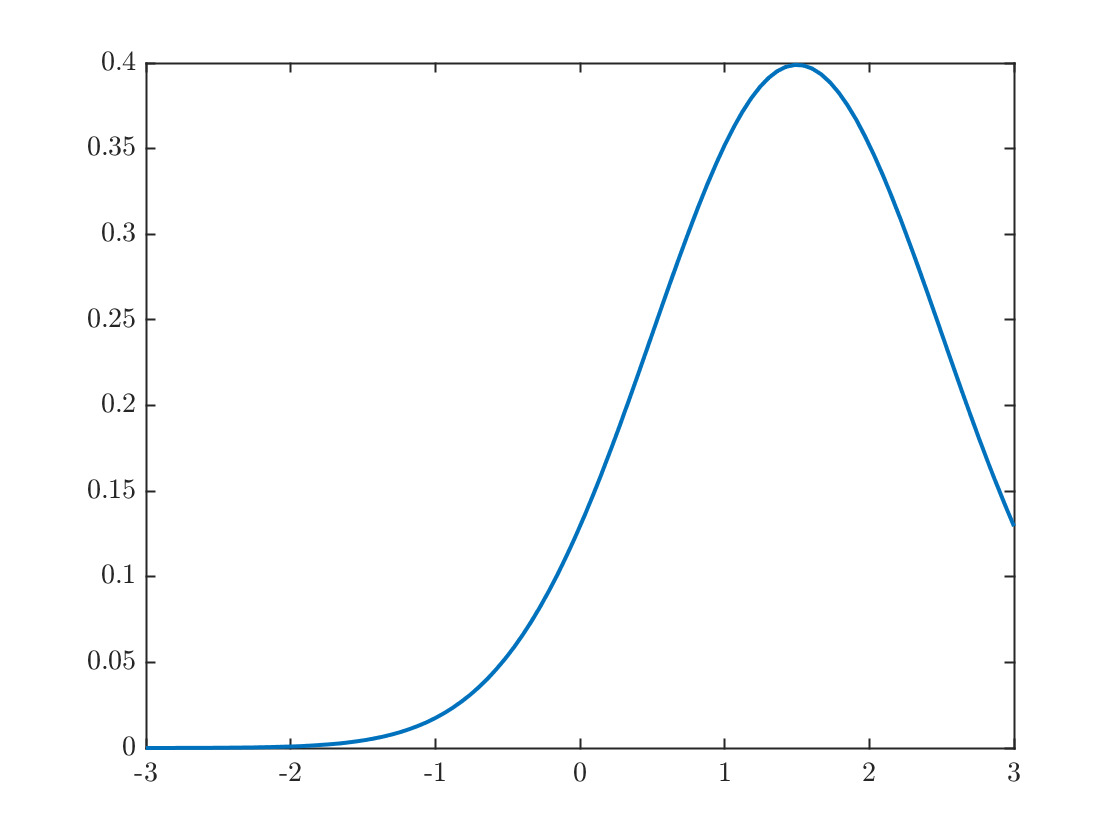A function can also have multiple outputs. For example
function [s,p]=sumprod(x1,x2)
s = x1+x2;
p = x1*x2;
end
Finally, simple functions with one output argumet can be defined quickly using the anonymous function syntax.
gaussian = @(x,mu,sigma) exp(-1/2 * ( (x-mu)/sigma).^2) / sigma / sqrt(2*pi);
This is called exactly like any other function. Anonymous functions can only have one output argument, so we can't write the sumprod example above this way.

### The Euler method

You learned in class the Euler method for approximating a solution to the differential equationGiven an initial condition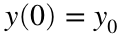, the method gives a sequence of approximations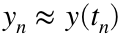defined by a reccurrence relation.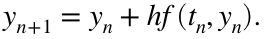where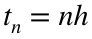and h is the time step chosen by the user.
Now we're ready for the real thing. Let's solve the problemThis has exact solution (which is easy to find because the ODE is separable)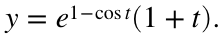tFinal = 2*pi;
N = 100;
h=tFinal/N;
t=linspace(0,tFinal,N+1); % type 'help linspace' to see how this works
y=zeros(1,N+1);
I want to point out the syntax used to define yExact in the above line. In particular the .* notation which is used for elementwise multiplication, since exp(1-cos(t)) and (1+t) are each vectors. You will need this to form the exact solution below, you will also need to use the .^ notation to compute an elementwise power.
y(1) = 1; % setting the initial condition
for n=1:N
y(n+1) = y(n) + h * f(t(n),y(n));
end
yExact=exactFunction(t);
plot(t,y,t,yExact,'--'); xlabel('t'); ylabel('y'); title('Look, ma! I solved an ODE in MATLAB!');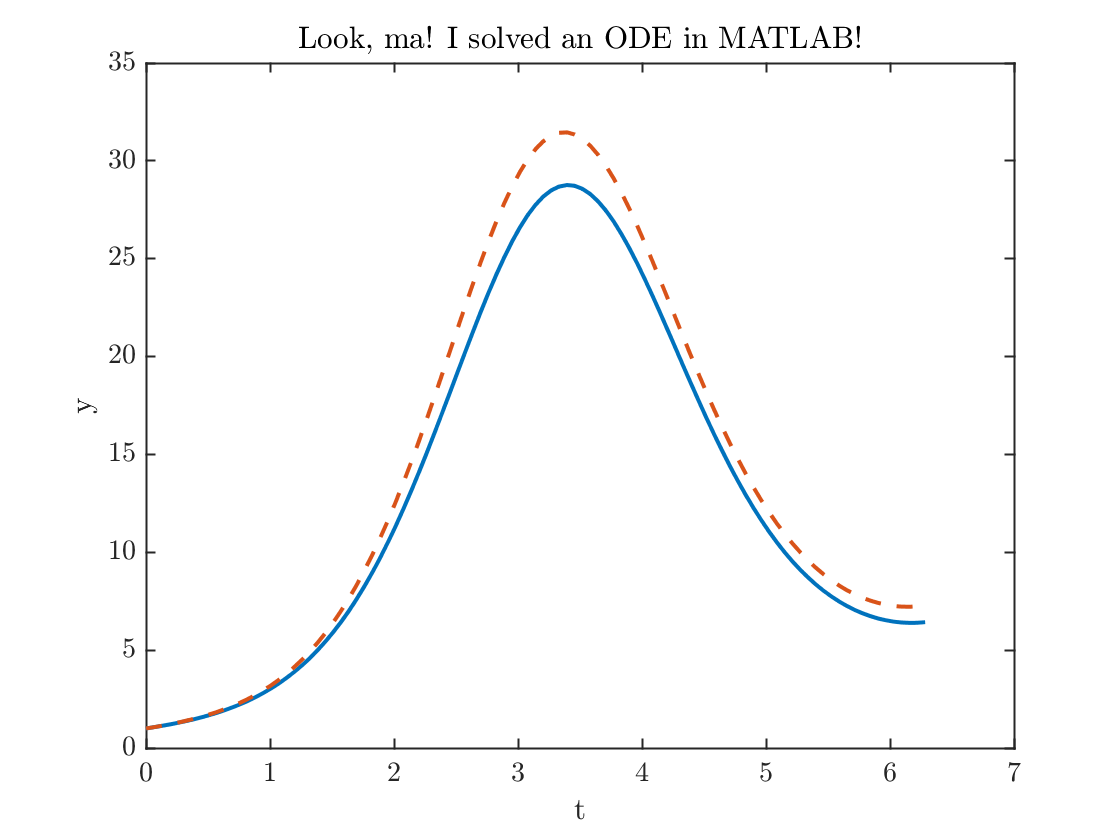error100= max(abs(y-yExact));
fprintf('The maximum error = %0.2f.\n',error100)
The maximum error = 2.85.
Some observations:
• I used the max function to evaluate the error.
• The code looks very neat because I use the call to the function f(t(n),y(n)) defined at the bottom of this file, instead of writing out the whole formula inside my loop.
• I used the fprintf command to format the output. Get help on this function by typing help fprintf at the MATLAB >> prompt.
That error is a little bit larger than I'd like, let's double the number of points and try again
N = 200;
h=tFinal/N;
t=linspace(0,tFinal,N+1); % type 'help linspace' to see how this works
y=zeros(1,N+1);
y(1) = 1; % setting the initial condition
for n=1:N
y(n+1) = y(n) + h * f(t(n),y(n));
end
yExact=exactFunction(t);
plot(t,y,t,yExact,'--')
xlabel('t'); ylabel('y'); title('Look, ma! I solved it even better!');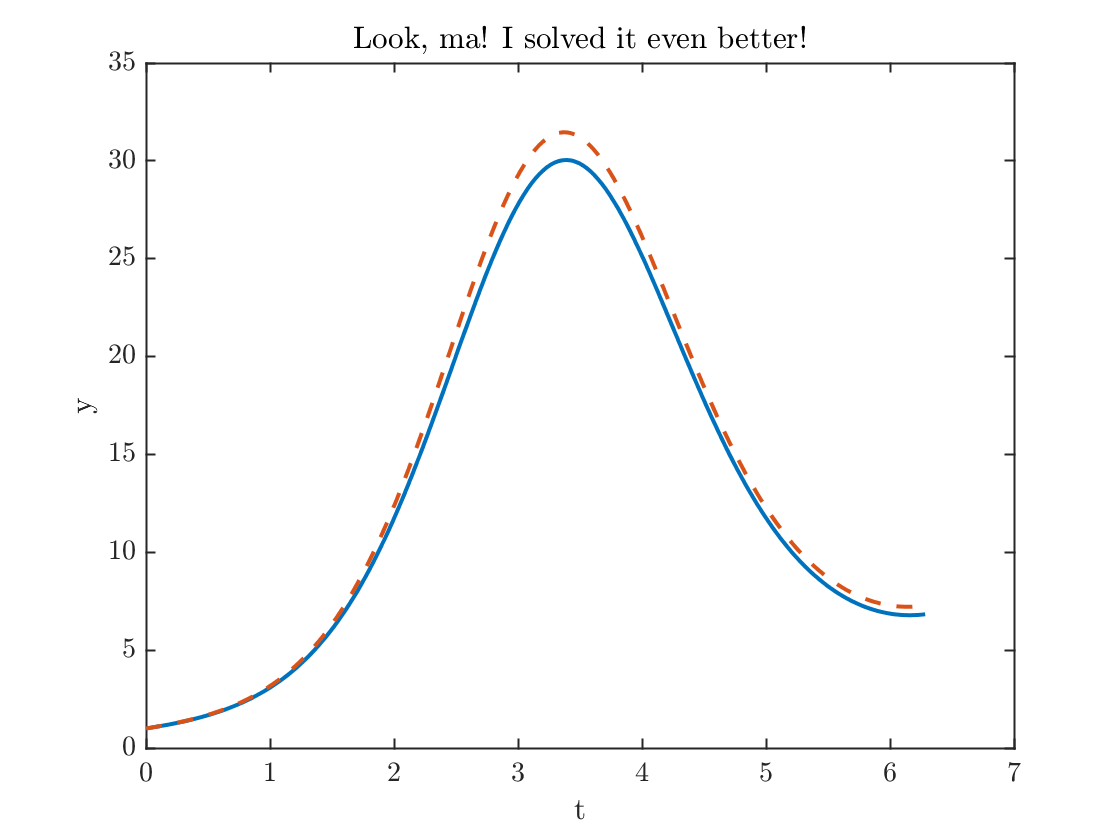error200= max(abs(y-yExact));
fprintf('The maximum error = %0.2f.\n',error200)
The maximum error = 1.49.
fprintf('The maximum error went down by a factor of %f.\n',error100/error200);
The maximum error went down by a factor of 1.907074.
Again, note how useful it is that I call the function f(t,y) in my loop instead of writing out the formula. This is good programming style, because if I decide to change the definition of f, I only have to change it one time, instead of twice.

### Functions used

Gaussian example
function y = gaussian(x,mu,sigma)
y = exp(-1/2 * ( (x-mu)/sigma).^2) / sigma / sqrt(2*pi);
end
Right hand side of ODE
function yprime=f(t,y)
yprime = (sin(t)+1/(1+t))*y;
end
Exact solution to the ODE
function x = exactFunction(t)
x= exp(1-cos(t)).*(1+t);
end